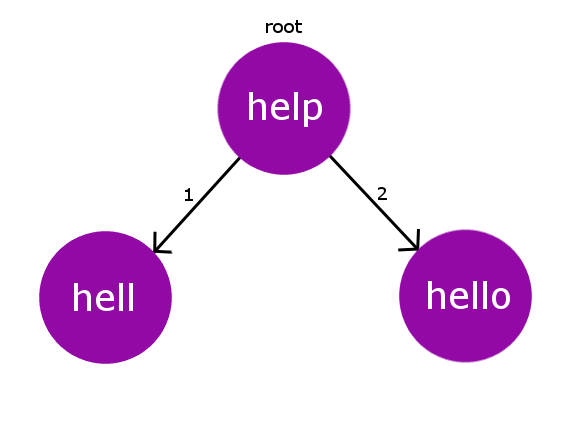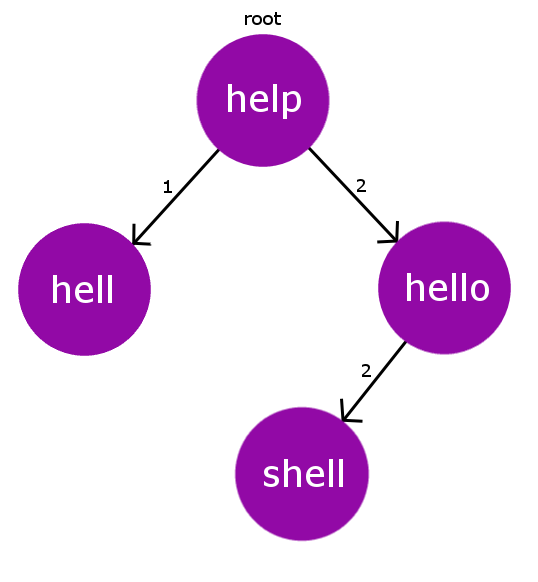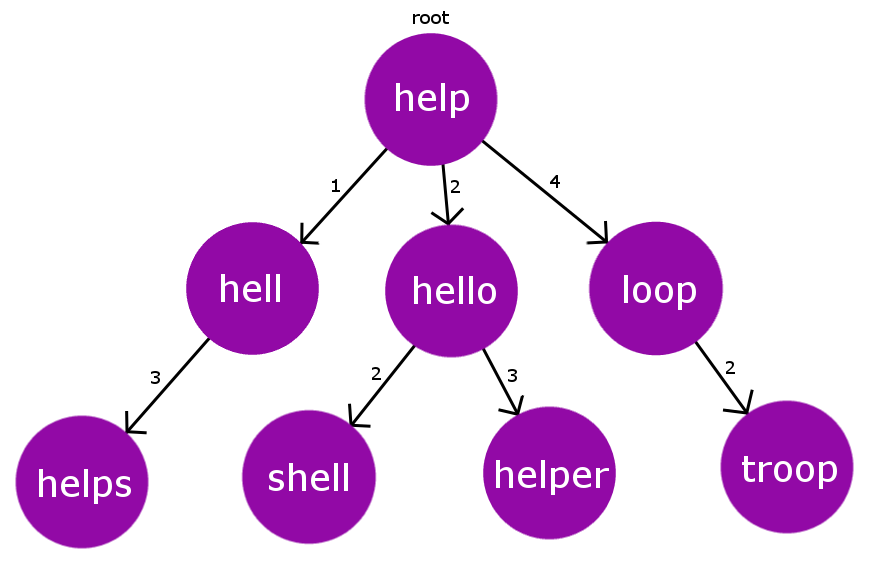# BK-Tree | Introduction & Implementation

• Difficulty Level : Hard
• Last Updated : 20 Apr, 2022

BK Tree or Burkhard Keller Tree is a data structure that is used to perform spell check based on Edit Distance (Levenshtein distance) concept. BK trees are also used for approximate string matching. Various auto correct feature in many software can be implemented based on this data structure.

```Pre-requisites : Edit distance Problem
Metric tree```

Let’s say we have a dictionary of words and then we have some other words which are to be checked in the dictionary for spelling errors. We need to have collection of all words in the dictionary which are very close to the given word. For instance if we are checking a word “ruk” we will have {“truck”,”buck”,”duck”,……}. Therefore, spelling mistake can be corrected by deleting a character from the word or adding a new character in the word or by replacing the character in the word by some appropriate one. Therefore, we will be using the edit distance as a measure for correctness and matching of the misspelled word from the words in our dictionary.
Now, let’s see the structure of our BK Tree. Like all other trees, BK Tree consists of nodes and edges. The nodes in the BK Tree will represent the individual words in our dictionary and there will be exactly the same number of nodes as the number of words in our dictionary. The edge will contain some integer weight that will tell us about the edit-distance from one node to another. Lets say we have an edge from node u to node v having some edge-weight w, then w is the edit-distance required to turn the string u to v.

Consider our dictionary with words : { “help” , “hell” , “hello”}. Therefore, for this dictionary our BK Tree will look like the below one.Every node in the BK Tree will have exactly one child with same edit-distance. In case, if we encounter some collision for edit-distance while inserting, we will then propagate the insertion process down the children until we find an appropriate parent for the string node.

Every insertion in the BK Tree will start from our root node. Root node can be any word from our dictionary.
For example, let’s add another word “shell” to the above dictionary. Now our Dict[] = {“help” , “hell” , “hello” , “shell”}. It is now evident that “shell” has same edit-distance as “hello” has from the root node “help” i.e 2. Hence, we encounter a collision. Therefore, we deal this collision by recursively doing this insertion process on the pre-existing colliding node.
So, now instead of inserting “shell” at the root node “help“, we will now insert it to the colliding node “hello“. Therefore, now the new node “shell” is added to the tree and it has node “hello” as its parent with the edge-weight of 2(edit-distance). Below pictorial representation describes the BK Tree after this insertion.So, till now we have understood how we will build our BK Tree. Now, the question arises that how to find the closest correct word for our misspelled word? First of all, we need to set a tolerance value. This tolerance value is simply the maximum edit distance from our misspelled word to the correct words in our dictionary. So, to find the eligible correct words within the tolerance limit, Naive approach will be to iterate over all the words in the dictionary and collect the words which are within the tolerance limit. But this approach has O(n*m*n) time complexity(n is the number of words in dict[], m is average size of correct word and n is length of misspelled word) which times out for larger size of dictionary.

Therefore, now the BK Tree comes into action. As we know that each node in BK Tree is constructed on basis of edit-distance measure from its parent. Therefore, we will directly be going from root node to specific nodes that lie within the tolerance limit. Lets, say our tolerance limit is TOL and the edit-distance of the current node from the misspelled word is dist. Therefore, now instead of iterating over all its children we will only iterate over its children that have edit distance in range

[dist-TOL , dist+TOL]. This will reduce our complexity by a large extent. We will discuss this in our time complexity analysis.
Consider the below constructed BK Tree.Let’s say we have a misspelled word “oop” and the tolerance limit is 2. Now, we will see how we will collect the expected correct for the given misspelled word.
Iteration 1: We will start checking the edit distance from the root node. D(“oop” -> “help”) = 3. Now we will iterate over its children having edit distance in range [ D-TOL , D+TOL ] i.e [1,5]
Iteration 2: Let’s start iterating from the highest possible edit distance child i.e node “loop” with edit distance 4.Now once again we will find its edit distance from our misspelled word. D(“oop”,”loop”) = 1.
here D = 1 i.e D <= TOL , so we will add “loop” to the expected correct word list and process its child nodes having edit distance in range [D-TOL,D+TOL] i.e [1,3]

Iteration 3: Now, we are at node “troop” . Once again we will check its edit distance from misspelled word . D(“oop”,”troop”)=2 .Here again D <= TOL , hence again we will add “troop” to the expected correct word list.

We will proceed the same for all the words in the range [D-TOL,D+TOL] starting from the root node till the bottom most leaf node. This, is similar to a DFS traversal on a tree, with selectively visiting the child nodes whose edge weight lie in some given range.
Therefore, at the end we will be left with only 2 expected words for the misspelled word “oop” i.e {“loop”, “troop”}

## C++

 `// C++ program to demonstrate working of BK-Tree``#include "bits/stdc++.h"``using` `namespace` `std;` `// maximum number of words in dict[]``#define MAXN 100` `// defines the tolerance value``#define TOL  2` `// defines maximum length of a word``#define LEN 10` `struct` `Node``{``    ``// stores the word of the current Node``    ``string word;` `    ``// links to other Node in the tree``    ``int` `next[2*LEN];` `    ``// constructors``    ``Node(string x):word(x)``    ``{``        ``// initializing next[i] = 0``        ``for``(``int` `i=0; i<2*LEN; i++)``            ``next[i] = 0;``    ``}``    ``Node() {}``};` `// stores the root Node``Node RT;` `// stores every Node of the tree``Node tree[MAXN];` `// index for current Node of tree``int` `ptr;` `int` `min(``int` `a, ``int` `b, ``int` `c)``{``    ``return` `min(a, min(b, c));``}` `// Edit Distance``// Dynamic-Approach O(m*n)``int` `editDistance(string& a,string& b)``{``    ``int` `m = a.length(), n = b.length();``    ``int` `dp[m+1][n+1];` `    ``// filling base cases``    ``for` `(``int` `i=0; i<=m; i++)``        ``dp[i] = i;``    ``for` `(``int` `j=0; j<=n; j++)``        ``dp[j] = j;` `    ``// populating matrix using dp-approach``    ``for` `(``int` `i=1; i<=m; i++)``    ``{``        ``for` `(``int` `j=1; j<=n; j++)``        ``{``            ``if` `(a[i-1] != b[j-1])``            ``{``                ``dp[i][j] = min( 1 + dp[i-1][j],  ``// deletion``                                ``1 + dp[i][j-1],  ``// insertion``                                ``1 + dp[i-1][j-1] ``// replacement``                              ``);``            ``}``            ``else``                ``dp[i][j] = dp[i-1][j-1];``        ``}``    ``}``    ``return` `dp[m][n];``}` `// adds curr Node to the tree``void` `add(Node& root,Node& curr)``{``    ``if` `(root.word == ``""` `)``    ``{``        ``// if it is the first Node``        ``// then make it the root Node``        ``root = curr;``        ``return``;``    ``}` `    ``// get its editDistance from the Root Node``    ``int` `dist = editDistance(curr.word,root.word);` `    ``if` `(tree[root.next[dist]].word == ``""``)``    ``{``        ``/* if no Node exists at this dist from root``         ``* make it child of root Node*/` `        ``// incrementing the pointer for curr Node``        ``ptr++;` `        ``// adding curr Node to the tree``        ``tree[ptr] = curr;` `        ``// curr as child of root Node``        ``root.next[dist] = ptr;``    ``}``    ``else``    ``{``        ``// recursively find the parent for curr Node``        ``add(tree[root.next[dist]],curr);``    ``}``}` `vector getSimilarWords(Node& root,string& s)``{``    ``vector < string > ret;``    ``if` `(root.word == ``""``)``       ``return` `ret;` `    ``// calculating editdistance of s from root``    ``int` `dist = editDistance(root.word,s);` `    ``// if dist is less than tolerance value``    ``// add it to similar words``    ``if` `(dist <= TOL) ret.push_back(root.word);` `    ``// iterate over the string having tolerance``    ``// in range (dist-TOL , dist+TOL)``    ``int` `start = dist - TOL;``    ``if` `(start < 0)``       ``start = 1;` `    ``while` `(start <= dist + TOL)``    ``{``        ``vector tmp =``             ``getSimilarWords(tree[root.next[start]],s);``        ``for` `(``auto` `i : tmp)``            ``ret.push_back(i);``        ``start++;``    ``}``    ``return` `ret;``}` `// driver program to run above functions``int` `main(``int` `argc, ``char` `const` `*argv[])``{``    ``// dictionary words``    ``string dictionary[] = {``"hell"``,``"help"``,``"shell"``,``"smell"``,``                           ``"fell"``,``"felt"``,``"oops"``,``"pop"``,``"oouch"``,``"halt"``                          ``};``    ``ptr = 0;``    ``int` `sz = ``sizeof``(dictionary)/``sizeof``(string);` `    ``// adding dict[] words on to tree``    ``for``(``int` `i=0; i match = getSimilarWords(RT,w1);``    ``cout << ``"similar words in dictionary for : "` `<< w1 << ``":\n"``;``    ``for` `(``auto` `x : match)``        ``cout << x << endl;` `    ``match = getSimilarWords(RT,w2);``    ``cout << ``"Correct words in dictionary for "` `<< w2 << ``":\n"``;``    ``for` `(``auto` `x : match)``        ``cout << x << endl;` `    ``return` `0;``}`

Output

```similar words in dictionary for : ops:
oops
pop
Correct words in dictionary for helt:
hell
help
shell
fell
felt
halt```

Time Complexity: It is quite evident that the time complexity majorly depends on the tolerance limit. We will be considering tolerance limit to be 2. Now, roughly estimating, the depth of BK Tree will be log n, where n is the size of dictionary. At every level we are visiting 2 nodes in the tree and performing edit distance calculation. Therefore, our Time Complexity will be O(L1*L2*log n), here L1 is the average length of word in our dictionary and L2 is the length of misspelled. Generally, L1 and L2 will be small.

References

This article is contributed by Nitish Kumar. If you like GeeksforGeeks and would like to contribute, you can also write an article using write.geeksforgeeks.org or mail your article to review-team@geeksforgeeks.org. See your article appearing on the GeeksforGeeks main page and help other Geeks.
Please write comments if you find anything incorrect, or you want to share more information about the topic discussed above.

My Personal Notes arrow_drop_up